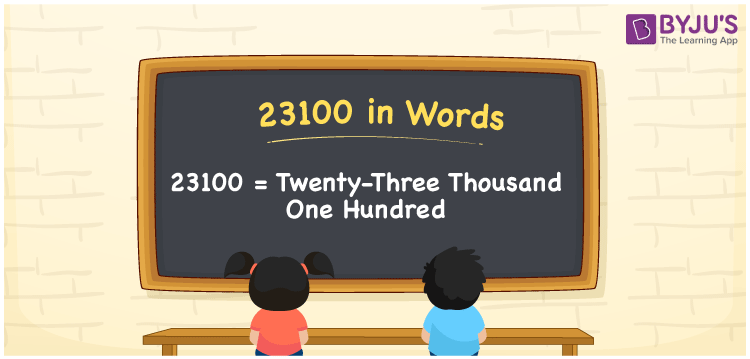# 23100 in words

23100 in words is written as Twenty Three Thousand One Hundred . In 23100, 2 has a place value of ten thousand, 3 is in the place value of thousand, 1 is in the place value of hundred. The article on Place Value gives more information. The number 23100 is used in expressions that relate to money, distance, length, Social media view, and many more. For example, “An Instagram post of a celebrity had Twenty Three Thousand One Hundred views within an hour.” Another example, “Rita’s Employee number is Twenty Three Thousand One Hundred.”

 23100 in words Twenty Three Thousand One Hundred Twenty Three Thousand One Hundred in Numbers 23100

## 23100 in English Words## How to Write 23100 in Words?

We can convert 23100 to words using a place value chart. The number 23100 has 5 digits, so let’s make a chart that shows the place value up to 5 digits.

 Ten thousand Thousands Hundreds Tens Ones 2 3 1 0 0

Thus, we can write the expanded form as:

2 × Ten thousand + 3 × Thousand + 1 × Hundred + 0 × Ten + 0 × One

= 2 × 10000 + 3 × 1000 + 1 × 100 + 0 × 10 + 0 × 1

= 23100.

= Twenty Three Thousand One Hundred.

23100 is the natural number that is succeeded by 23099 and preceded by 23101.

23100 in words – Twenty Three Thousand One Hundred.

Is 23100 an odd number? – No.

Is 23100 an even number? – Yes.

Is 23100 a perfect square number? – No.

Is 23100 a perfect cube number? – No.

Is 23100 a prime number? – No.

Is 23100 a composite number? – Yes.

## Solved Example

1. Write the number 23100 in expanded form

Solution: 2 x 10000 + 3 x 1000 + 1 x 100 + 0 x 10 + 0 x 1

Or Just 2 x 10000 + 3 x 1000 + 1 x 100

We can write 23100 = 20000 + 3000 + 100 + 0 + 0

= 2 x 10000 + 3 x 1000 + 1 x 100 + 0 x 10 + 0 x 1.

## Frequently Asked Questions on 23100 in words

Q1

### How to write the number 23100 in words?

23100 in words is written as Twenty Three Thousand One Hundred.
Q2

### Is 23100 divisible by 3?

Yes. 23100 is divisible by 3.
Q3

### Is 23100 divisible by 10?

Yes. 23100 is divisible by 10.